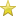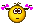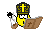Weisheiten oder so^^^^Sunburst⚧ Präklassische TSBeiträge: 3.537 Themen: 19 Registriert seit: Feb 2018 Beziehung zu Forum/Thema: Post-OP Land: Indien RE: Weisheiten oder so^^^^ Beitrag #43 21.11.2018, 23:24 यैरेव पतनं द्रव्यैः सिद्धिस्तैरेव चोदिता yaireva patanaṃ dravyaiḥ siddhistaireva coditā "Success is attained by those very things which lead to [one‛s] fall" (Kulārṇava Tantra 5.48) Προσποιοῦμαι παίζεινNachrichten in diesem Thema Weisheiten oder so^^^^ - von Inselmaus - 01.11.2012, 20:50 RE: Weisheiten oder so^^^^ - von Inselmaus - 04.11.2012, 14:15 RE: Weisheiten oder so^^^^ - von jasminchen - 04.11.2012, 23:13 RE: Weisheiten oder so^^^^ - von jasminchen - 04.11.2012, 23:40 RE: Weisheiten oder so^^^^ - von Sarah-Michelle - 05.11.2012, 00:47 RE: Weisheiten oder so^^^^ - von Inselmaus - 20.11.2012, 15:12 RE: Weisheiten oder so^^^^ - von NiAypa - 28.11.2012, 16:07 RE: Weisheiten oder so^^^^ - von Ulli - 28.11.2012, 18:57 RE: Weisheiten oder so^^^^ - von Shabana - 28.11.2012, 19:49 RE: Weisheiten oder so^^^^ - von Angelika - 29.11.2012, 11:43 RE: Weisheiten oder so^^^^ - von Inselmaus - 29.11.2012, 13:48 RE: Weisheiten oder so^^^^ - von Alexandra - 29.11.2012, 21:00 RE: Weisheiten oder so^^^^ - von Ulli - 29.11.2012, 21:53 RE: Weisheiten oder so^^^^ - von Alexandra - 29.11.2012, 22:06 RE: Weisheiten oder so^^^^ - von Ulli - 29.11.2012, 22:41 RE: Weisheiten oder so^^^^ - von Ulli - 29.11.2012, 22:48 RE: Weisheiten oder so^^^^ - von Bonita - 30.11.2012, 11:29 RE: Weisheiten oder so^^^^ - von Ulli - 30.11.2012, 12:37 RE: Weisheiten oder so^^^^ - von jasminchen - 23.12.2012, 05:40 RE: Weisheiten oder so^^^^ - von Eva_Tg - 26.12.2012, 04:53 RE: Weisheiten oder so^^^^ - von Sopherl - 28.12.2012, 18:01 RE: Weisheiten oder so^^^^ - von Sinead Euphony - 29.12.2012, 18:30 RE: Weisheiten oder so^^^^ - von Miriam - 30.12.2012, 00:09 RE: Weisheiten oder so^^^^ - von Bonita - 11.03.2013, 14:33 RE: Weisheiten oder so^^^^ - von Damb - 11.03.2013, 18:21 RE: Weisheiten oder so^^^^ - von Theo34 - 12.03.2013, 19:33 RE: Weisheiten oder so^^^^ - von Carina_in_Graz - 18.03.2013, 02:54 RE: Weisheiten oder so^^^^ - von Ulli - 18.03.2013, 20:47 RE: Weisheiten oder so^^^^ - von wildehilde - 18.03.2013, 23:24 RE: Weisheiten oder so^^^^ - von Ulli - 20.03.2013, 10:49 RE: Weisheiten oder so^^^^ - von wildehilde - 20.03.2013, 23:07 RE: Weisheiten oder so^^^^ - von Ulli - 23.03.2013, 13:02 RE: Weisheiten oder so^^^^ - von Sabrina Crossdress - 21.03.2013, 16:21 RE: Weisheiten oder so^^^^ - von GeminiStigma - 27.04.2015, 17:02 RE: Weisheiten oder so^^^^ - von FreedomMusic - 06.05.2015, 13:32 RE: Weisheiten oder so^^^^ - von Madleine - 08.08.2015, 20:33 RE: Weisheiten oder so^^^^ - von Ein Mäderl - 08.08.2015, 20:49 RE: Weisheiten oder so^^^^ - von Madleine - 17.08.2015, 22:11 RE: Weisheiten oder so^^^^ - von ConfusedRaven - 18.08.2015, 22:21 RE: Weisheiten oder so^^^^ - von Ирина - 23.02.2017, 22:43 RE: Weisheiten oder so^^^^ - von Ирина - 01.03.2017, 00:35 RE: Weisheiten oder so^^^^ - von Sunburst - 21.11.2018, 15:20 RE: Weisheiten oder so^^^^ - von Sunburst - 21.11.2018, 23:24

Gehe zu: